# Floating Point Number Confusion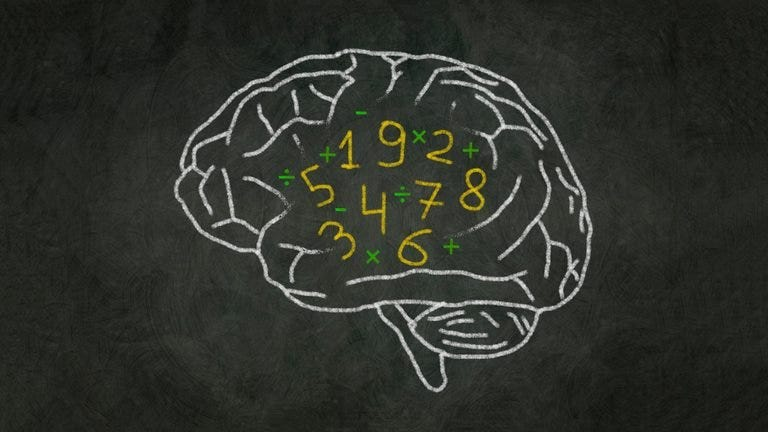Source (https://www.rd.com/article/what-is-mental-math-tricks/)

First of all, I will ask a small question. How many time the below-mentioned for-loop will execute;

Most of you may say it will iterate 100 or 99. But actually, the for-loop will run indefinitely without stopping. To understand that, we need to see how the floating-point behaves inside the computers. The IEEE has introduced a Standard for the Floating Point Arithmetics (IEEE 754) and it is a technical standard for floating-point arithmetic established in 1985 by the Institute of Electrical and Electronics Engineers (IEEE).

`Output of the above code segment :10.09.99.89.7000000000000019.6000000000000019.5000000000000029.4000000000000029.3000000000000029.2000000000000039.1000000000000039.0000000000000048.9000000000000048.8000000000000048.7000000000000058.6000000000000058.5000000000000058.4000000000000068.3000000000000068.2000000000000068.1000000000000078.0000000000000077.90000000000000757.8000000000000087.7000000000000087.60000000000000857.5000000000000097.4000000000000097.300000000000017.200000000000017.100000000000017.0000000000000116.9000000000000116.8000000000000116.7000000000000126.6000000000000126.5000000000000126.4000000000000136.3000000000000136.20000000000001356.1000000000000146.0000000000000145.9000000000000155.8000000000000155.7000000000000155.6000000000000165.5000000000000165.4000000000000165.3000000000000175.2000000000000175.1000000000000175.0000000000000184.9000000000000184.80000000000001854.7000000000000194.6000000000000194.50000000000001954.400000000000024.300000000000024.2000000000000214.1000000000000214.0000000000000213.90000000000002123.8000000000000213.7000000000000213.6000000000000213.5000000000000213.4000000000000213.30000000000002073.20000000000002063.10000000000002053.00000000000002042.90000000000002032.80000000000002032.700000000000022.600000000000022.500000000000022.400000000000022.300000000000022.20000000000001972.10000000000001962.00000000000001951.90000000000001951.80000000000001941.70000000000001931.60000000000001921.5000000000000191.4000000000000191.3000000000000191.20000000000001881.10000000000001871.00000000000001870.90000000000001870.80000000000001870.70000000000001870.60000000000001870.50000000000001880.40000000000001880.30000000000001880.20000000000001880.10000000000001881.8790524691780774E-14-0.09999999999998122-0.19999999999998122-0.2999999999999812-0.39999999999998126-0.49999999999998124-0.5999999999999812-0.6999999999999812-0.7999999999999812-0.8999999999999811-0.9999999999999811-1.0999999999999812-1.1999999999999813-1.2999999999999814-1.3999999999999815......// and like so it will continue indefinitely`

As all of you know, computers will convert any number to binary(bits) before doing any kind of arithmetic operation. Then perform addition and subtraction on those converted binary data to obtain results of the arithmetic operations. According to the above standard, the floating-point number will be categorized based on precision as single, double, and long double, as shown below. Most importantly, a given floating-point number will divide into three parts as Sign, Exponent, and Mantissa. Each part has a designated maximum number of bits that can be used to represent a floating-point number at the machine level, as shown below.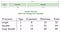IEEE 754 Floating-Point Standard

# Decimal to Binary Conversion Process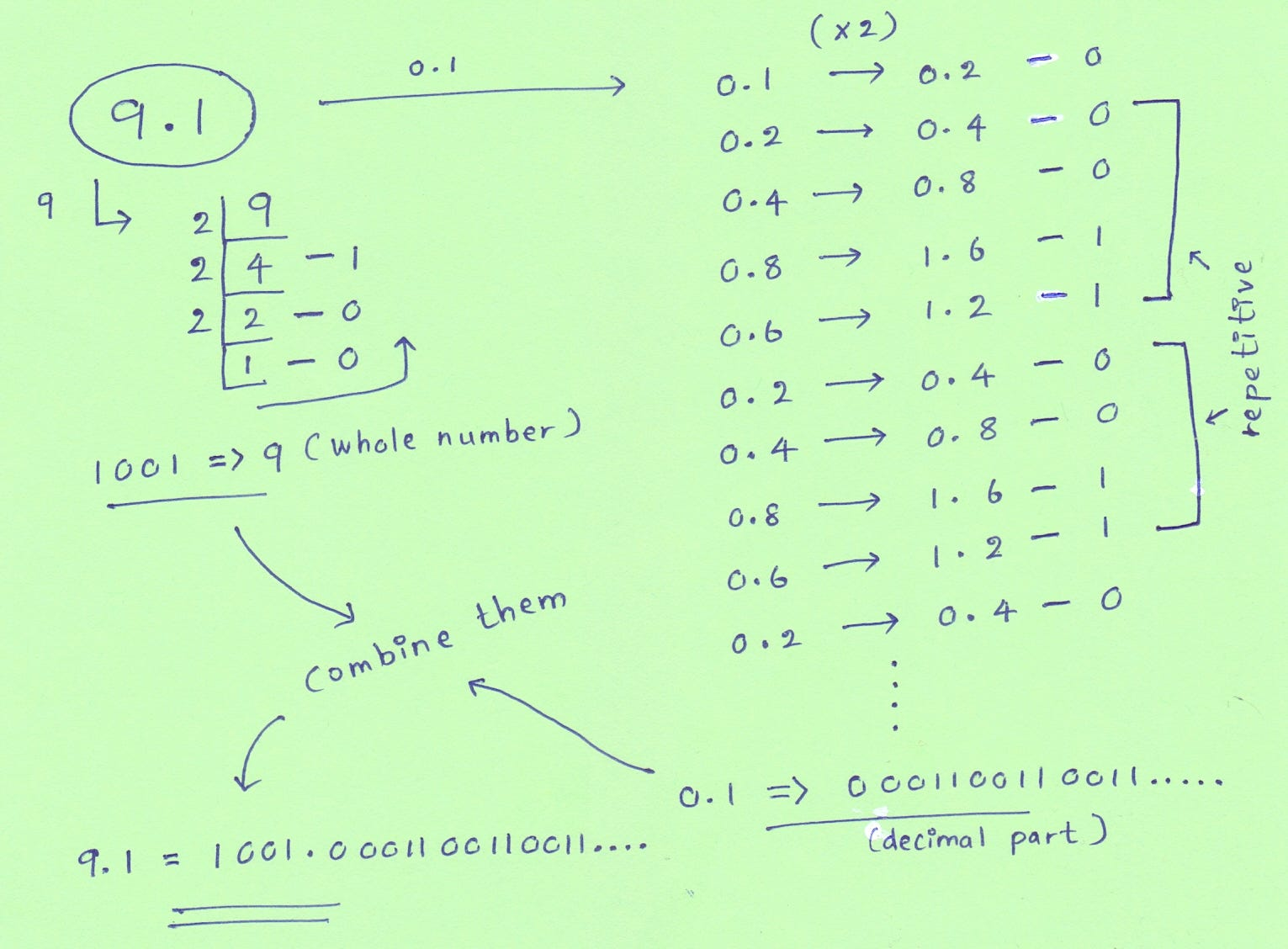Figure 1: Conversion of 9.1 to Binary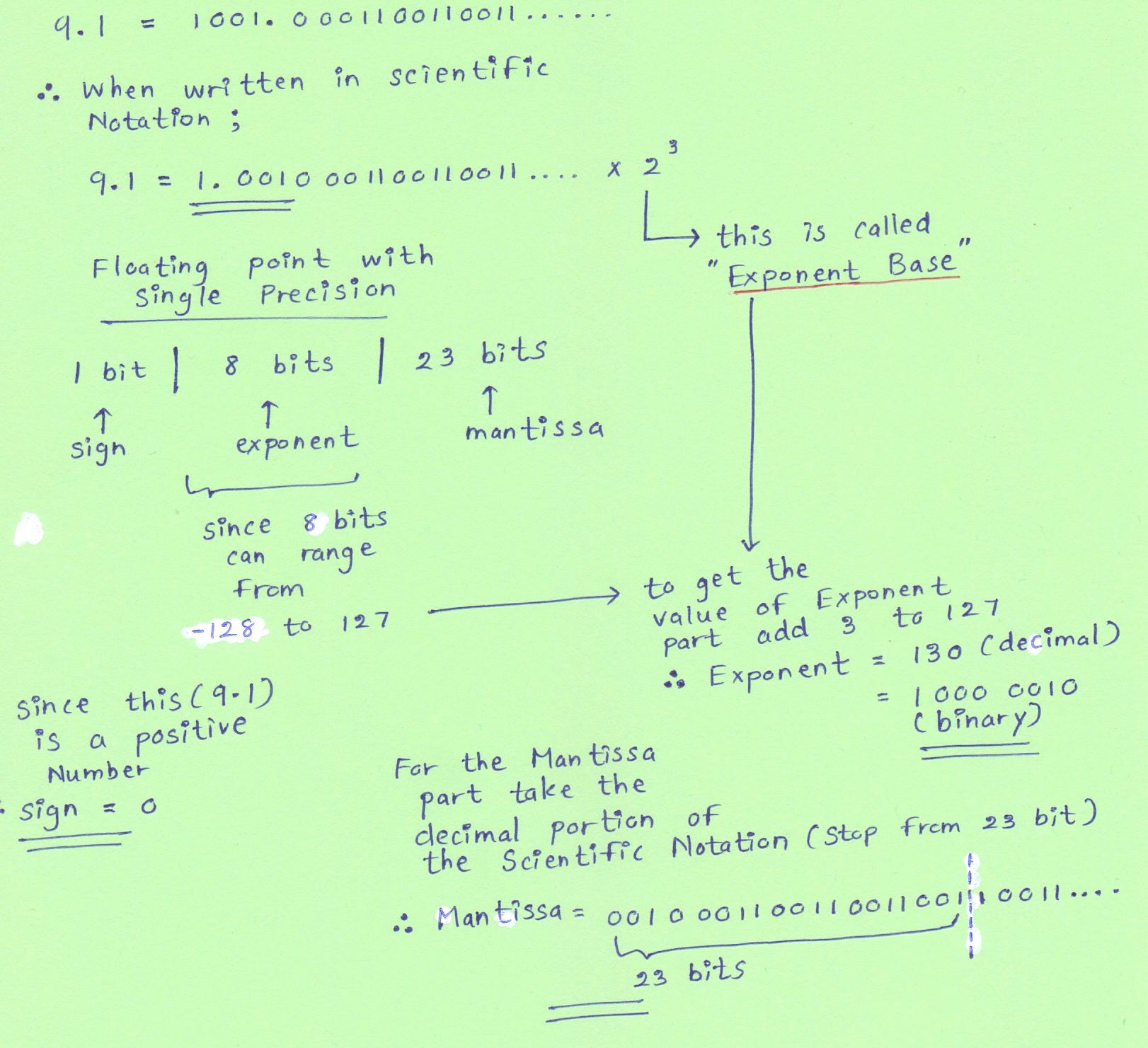Figure 2: Scientific Notation, Sign value, Exponent value, and Manytissa value

We will consider 9.1 as a single-precision number, but the process is the same for other precision numbers as well. As shown in figure 1, when converting 9.1 to binary first convert 9 to binary and it will be 1001. Then convert the 0.1 to binary it will be 00011001100110011…. recurring binary number. After that, write the binary format of the 9.1 in the scientific nation, and it will be 1.00100011001100110011…. x ²³. The ²³ is referred to as Exponent Base and adds that power 3 to the 127 get the Exponent Bits (since it ranges from -128 to 127) of floating-point number representation as shown in figure 2. Since this is a positive number, it will be 0 at Sign Bit. Then exclude the 1 in the scientific notation of the 9.1 and take the decimal part and store it in Mantissa Bits (Only 23 bits ignore the rest). So the finalize floating-point number representation of 9.1 according to the IEEE 754 is 01000001000100011001100110011001, as shown in figure 3.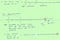Figure 3: Approximation in the Mantissa Bits

Now comes the tricky part, the Mantissa section is 00100011001100110011001….. when storing on computer memory, it will have only have 23 bits. What happened here is when storing only 23 bits from this recurring decimal, it will look whether the 24th bit is 1 or 0. If it is 1, add 1 bit to the 23rd-bit position. If it is 0, leave it as it is. Since in binary representation of 9.1 have 1 bit at the 24th-bit position, it will add 1 bit to the 23rd-bit position. So our final IEEE 754 floating-point number representation would be 01000001000100011001100110011011. As shown in figure 3, I have highlighted the difference in IEEE 754 floating-point number representation due to approximation happens in the Mantissa Section.

# Binary to Decimal Conversion Process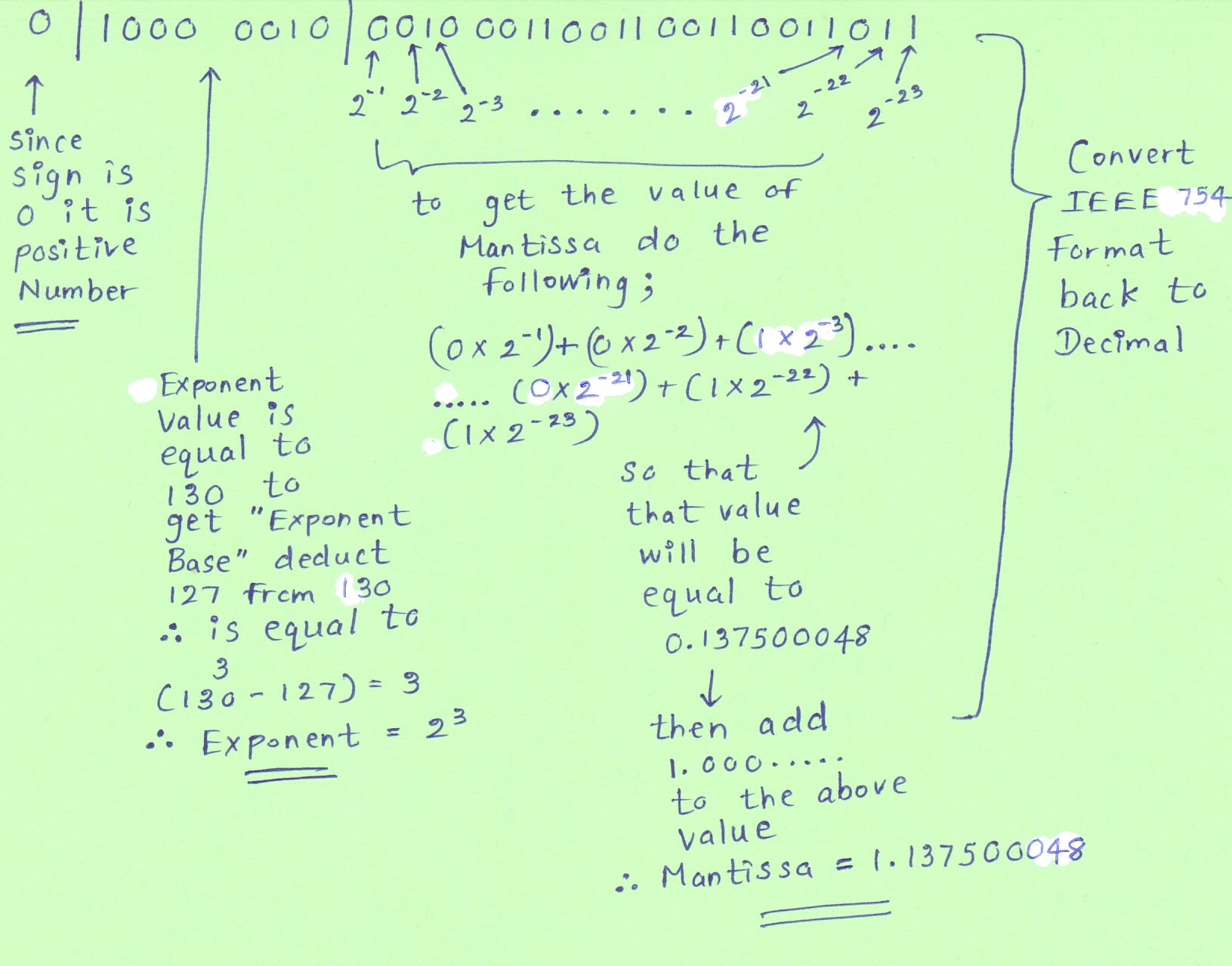Figure 4: Converting Binary back to Decimal values

So the binary representation of 9.1 according to IEEE 754 is 01000001000100011001100110011011. When converting this binary format to decimal format again, since the Sign Bit is 0 number will be positive. The Exponent Bits have 130 in decimal value, so to get the Exponent Base, deduct 127 from that, as shown in figure 4. Therefore Converted Exponent part will be ²³. Then we can convert the Mantissa Bits to decimal, as shown in figure 4, and add 1 to it since we exclude bits left to the decimal point in scientific notations. So the Mantissa decimal value is 1.137500048. After that, multiply the converted Exponent and Mantissa parts, so now the finalized result when convert binary back to decimal value will be 9.10000048, as shown in figure 5.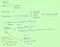Figure 5: additional value getting from back and forth binary to decimal conversions

So you can see when we convert back and forth from decimal to binary and binary to decimal inside the computers, we will get some additional values due to IEEE 754 standard approximation happen in the Mantissa section’s last bit. As a result of that, when we do subtraction continuously from the floating-point numbers in a computer, it will not hit zero exactly but go beyond zero to the negative side because of extra values generated from rounding off happen in the mantissa last bit, which is called Floating-Point Rounding Error. Now you can understand why the for-loop mention at the beginning of the blog will continue to execute indefinitely.

# BigDecimal in Java

To avoid this floating-point issue, java has introduced a class called BigDecimal. BigDecimal is an arbitrary-precision signed immutable decimal number. BigDecimal consists of two parts as Unscaled value and Scale value. For example, if we consider the 10.342 BigDecimal number, it has an unscaled value of 10342, and the value of scale is 3.

• Unscaled Value: An arbitrary precision integer
• Scale: A 32-bit integer representing the number of digits to the right of the decimal point

When we are, dealing with high precision arithmetic or when we need control over scaling and rounding off behavior, BigDemical will be very helpful. One such example is calculations involving financial transactions. There are several ways to create Big Decimals using Strings, character arrays, integers, and long, as shown below.

Also, we can perform arithmetic operations such as addition, subtraction, multiplication, and division using BigDecimal, as shown below. If we need to perform a comparison on BigDecimal objects, we can use the compareTo operator. Since BigDecimal is immutable, arithmetic operations do not modify the existing objects. They will return new objects. To get to know about more operations you can perform on BigDecimal objects, visit https://www.baeldung.com/java-bigdecimal-biginteger.

Now, let’s rewrite the for-loop mentioned at the beginning of the article using BigDecimal and see we will get the output as we are expecting. I have used the compareTo operator to perform the comparison on BigDecimal objects.

`Now the output of the above code segment is similar to what we all think:109.99.89.79.69.59.49.39.29.19.08.98.88.78.68.58.48.38.28.18.07.97.87.77.67.57.47.37.27.17.06.96.86.76.66.56.46.36.26.16.05.95.85.75.65.55.45.35.25.15.04.94.84.74.64.54.44.34.24.14.03.93.83.73.63.53.43.33.23.13.02.92.82.72.62.52.42.32.22.12.01.91.81.71.61.51.41.31.21.11.00.90.80.70.60.50.40.30.20.1`

# References

For further more clarification check these resources;

Software Engineer at Virtusa, Graduate of Sri Lanka Institute of Information Technology (SLIIT).

Software Engineer at Virtusa, Graduate of Sri Lanka Institute of Information Technology (SLIIT).